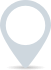# Weighted average cost of capital calculator

The Weighted Average Cost of Capital (WACC) is a financial metric that represents the average cost of financing a company’s assets, considering both debt and equity components.15%
£
.00
8%
£
.00
0%

This calculator is intended for illustration purposes only and exact payment terms should be agreed with a lender before taking out a loan.

Weighted average cost of capital

-

## How to calculate weighted average cost of capital

To calculate the weighted average cost of capital:

1. Determine the market value of equity (E) and the market value of debt (D). Add these two values to find the total value of the firm (V).
2. Determine the cost of equity (Re) and the cost of debt (Rd). These are the required rates of return on equity and debt, respectively.
3. Find the corporate tax rate (Tc) applicable to the company.
4. Plug the values into the formula to calculate the WACC.

### Clever finance tips and the latest news

#### delivered to your inbox, every week